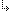- 4.45.2.29. -4. Standard Units4.45. Math - Extended Mathematics unit4.45.2. Math Unit Procedures and Functions4.45.2.29. FMod function

## 4.45.2.29. FMod function

Targets: MS-DOS, OS/2, Win32

Math Unit

Calculates the floating-point remainder.

Declaration:
`function Fmod(X, Y: Extended): Extended;`
Remarks:
The Fmod function calculates the floating-point remainder F of X / Y such that X = i * Y  + F, where I is an integer, F has the same sign as X, and the absolute value of F is less than the absolute value of Y.

- 4.45.2.29. -